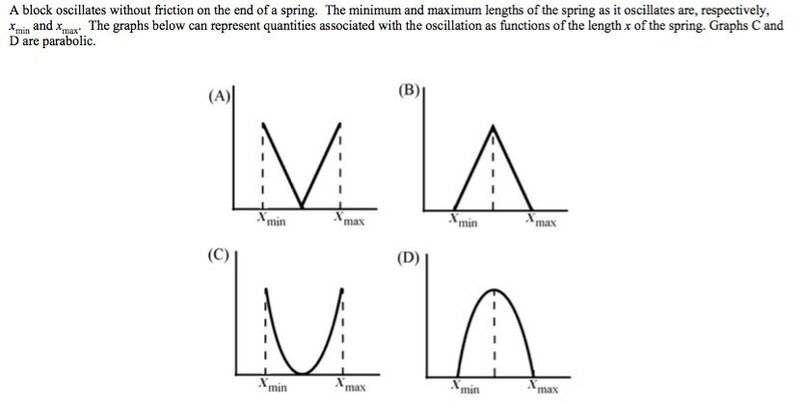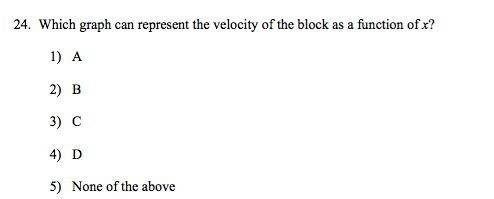# Graphs relating to simple harmonic motion

RoboNerd

## Homework StatementNone.

## The Attempt at a Solution

Hi everyone. Apparently 5 is the right answer, although I chose D.

Could anyone please weigh in with their thoughts about why 5 is right and my answer is apparently wrong?

Thanks!

Homework Helper
Gold Member

Physics-Tutor
Velocity can also be negative...

Homework Helper
Gold Member
Velocity can also be negative...
True, but that does not rule out any of the graphs. There is no claim that the graph represents an entire cycle. There is a better reason for choosing 5. What would the graph of velocity actually look like?

RoboNerd
Hi everyone, robonerd is back, of course.

I know that at xmin and max, the velocity [with kinetic energy] = 0 instantaneously. Thus, I narrow down to B and D.
However, I know that with a variable spring force giving a variable acceleration, I will not have the velocity changing in a linear manner [constant acceleration with constant slope], so I rule out B. D is thus a potential answer. Why is D wrong?

Homework Helper
Gold Member
Hi everyone, robonerd is back, of course.

I know that at xmin and max, the velocity [with kinetic energy] = 0 instantaneously. Thus, I narrow down to B and D.
However, I know that with a variable spring force giving a variable acceleration, I will not have the velocity changing in a linear manner [constant acceleration with constant slope], so I rule out B. D is thus a potential answer. Why is D wrong?
Can you write an equation relating velocity and x?

RoboNerd
Yes. Using conservation of energy I have:

( 1 / 2 ) * k * A^2 = a constant value = (1 / 2) * m * v^2 + ( 1/ 2) * k * x^2